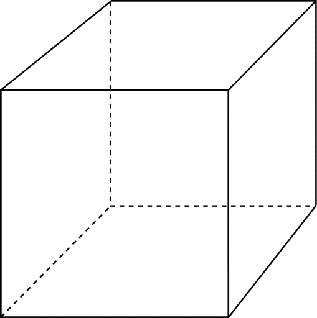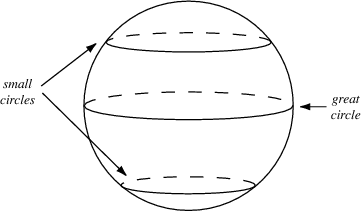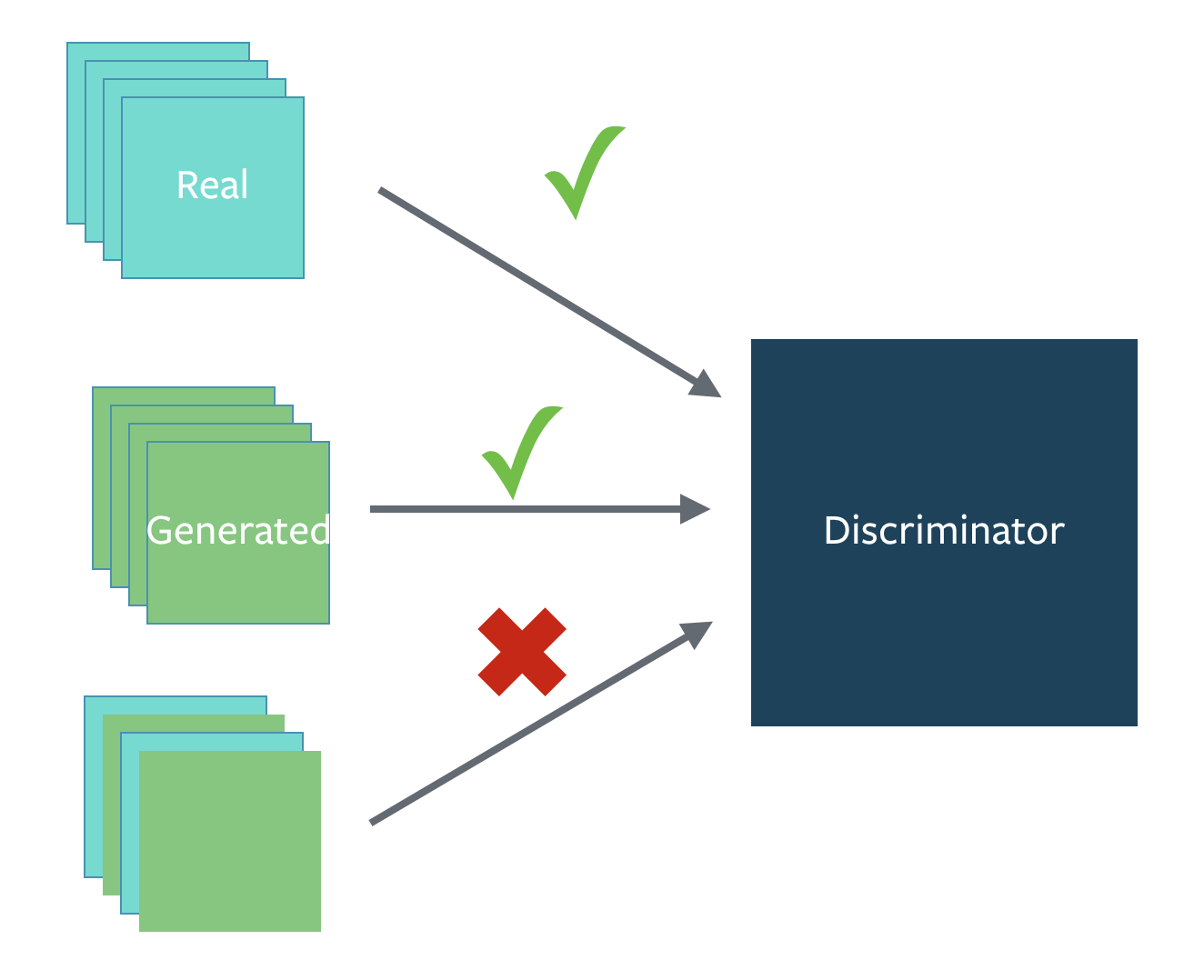# taylover2016 / ganhacks

starter from "How to Train a GAN?" at NIPS2016

Geek Repo

Github PK Tool

(this list is no longer maintained, and I am not sure how relevant it is in 2020)

# How to Train a GAN? Tips and tricks to make GANs work

While research in Generative Adversarial Networks (GANs) continues to improve the fundamental stability of these models, we use a bunch of tricks to train them and make them stable day to day.

Here are a summary of some of the tricks.

Here's a link to the authors of this document

If you find a trick that is particularly useful in practice, please open a Pull Request to add it to the document. If we find it to be reasonable and verified, we will merge it in.

## 1. Normalize the inputs

• normalize the images between -1 and 1
• Tanh as the last layer of the generator output

## 2: A modified loss function

In GAN papers, the loss function to optimize G is `min (log 1-D)`, but in practice folks practically use `max log D`

• because the first formulation has vanishing gradients early on
• Goodfellow et. al (2014)

In practice, works well:

• Flip labels when training generator: real = fake, fake = real

## 3: Use a spherical Z

• Dont sample from a Uniform distribution• Sample from a gaussian distribution## 4: BatchNorm

• Construct different mini-batches for real and fake, i.e. each mini-batch needs to contain only all real images or all generated images.
• when batchnorm is not an option use instance normalization (for each sample, subtract mean and divide by standard deviation).## 5: Avoid Sparse Gradients: ReLU, MaxPool

• the stability of the GAN game suffers if you have sparse gradients
• LeakyReLU = good (in both G and D)
• For Downsampling, use: Average Pooling, Conv2d + stride
• For Upsampling, use: PixelShuffle, ConvTranspose2d + stride

## 6: Use Soft and Noisy Labels

• Label Smoothing, i.e. if you have two target labels: Real=1 and Fake=0, then for each incoming sample, if it is real, then replace the label with a random number between 0.7 and 1.2, and if it is a fake sample, replace it with 0.0 and 0.3 (for example).
• Salimans et. al. 2016
• make the labels the noisy for the discriminator: occasionally flip the labels when training the discriminator

## 7: DCGAN / Hybrid Models

• Use DCGAN when you can. It works!
• if you cant use DCGANs and no model is stable, use a hybrid model : KL + GAN or VAE + GAN

## 8: Use stability tricks from RL

• Experience Replay
• Keep a replay buffer of past generations and occassionally show them
• Keep checkpoints from the past of G and D and occassionaly swap them out for a few iterations
• All stability tricks that work for deep deterministic policy gradients
• See Pfau & Vinyals (2016)

## 9: Use the ADAM Optimizer

• See Radford et. al. 2015
• Use SGD for discriminator and ADAM for generator

## 10: Track failures early

• D loss goes to 0: failure mode
• check norms of gradients: if they are over 100 things are screwing up
• when things are working, D loss has low variance and goes down over time vs having huge variance and spiking
• if loss of generator steadily decreases, then it's fooling D with garbage (says martin)

## 11: Dont balance loss via statistics (unless you have a good reason to)

• Dont try to find a (number of G / number of D) schedule to uncollapse training
• It's hard and we've all tried it.
• If you do try it, have a principled approach to it, rather than intuition

For example

``````while lossD > A:
train D
while lossG > B:
train G
``````

## 12: If you have labels, use them

• if you have labels available, training the discriminator to also classify the samples: auxillary GANs

## 13: Add noise to inputs, decay over time

• Add some artificial noise to inputs to D (Arjovsky et. al., Huszar, 2016)
• adding gaussian noise to every layer of generator (Zhao et. al. EBGAN)
• Improved GANs: OpenAI code also has it (commented out)

## 14: [notsure] Train discriminator more (sometimes)

• especially when you have noise
• hard to find a schedule of number of D iterations vs G iterations

## 15: [notsure] Batch Discrimination

• Mixed results

## 16: Discrete variables in Conditional GANs

• Use an Embedding layer